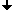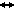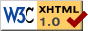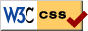Nonlinear Dynamics at the Free University Berlin

Winter 2014

# Seminar Advanced Topics in Nonlinear Dynamics

Prof. Dr. Bernold Fiedler, PD Dr. Pavel Gurevich, PD Dr. Stefan Liebscher, Dr. Juliette Hell

## Program

 Oct 16, 2014 Informal Meeting Planning Suggestions of topics and schedule Oct 30, 2014 Maxime Percie du Sert (Université Paris Sud) Smale-Sard-Thom transversality: an introduction and applications to networks tba Nov 6, 201414h15-17h Helmut Friedrich (AEI Potsdam) Geometric Asymptotics and Beyond We consider solutions to Einstein's field equations with positive cosmological constant and compact space sections. It has been known for a while that a large class of vacuum solutions or solutions with zero rest-mass matter fields admits smooth conformal extensions to future time-like infinity and beyond. This partly motivated the conformal cyclic cosmology model recently proposed by R. Penrose. Because of some unsolved question arising in this model we analyzed the behaviour of matter fields with non-vanishing rest-mass at time-like infinity. Somewhat unexpectedly it turned out that under certain assumptions some such fields do admit smooth conformal extensions. We discuss the general background and the arguments which led to this result, present the system of ODE's obtained under suitable symmetry assumptions, and discuss some of the open problems. Mark Curran (FU Berlin) Presentation Master thesis 16h15 - 17h Nov 13, 201414h15-16h30 Markus Mittnenzweig(WIAS Berlin) Presentation Master thesis - Entropy Production Inequalities for Heat Equations Entropy production inequalities are a good method to prove convergence of solutions of heat equations to asymptotic distributions. In this work we will use entropies that are motivated from thermodynamics and derive entropy production inequalities for them. As a first application, we use these inequalities to prove exponential convergence of solutions of nonlinear diffusion equations. Hannes Stuke (FU Berlin) Analytic continuation beyond blow-up - Main Idea and formal calculations (Part 1) In my talk I will introduce a class of reaction-diffusion equations for which I will present the main idea to show, that analytic continuation onto the real axis after blow-up is possible. I will support the idea by formal calculations and furthermore I will draw some conclusions about blow-up rate and blow-up set from the analytic continued solutions. Nov 20, 2014 Anna Karnauhova(FU Berlin) Meander - Results and Overview Let M denote a rainbow meander with N arches. It is known that for N < 4 there is a closed gcd-expression counting the number of connected components of M. We will prove that for N > 3 there are no homogeneous polynomials f1, ..., fm of arbitrary degree such that the number of connected components equals to the greatest common divisor of f1, ..., fm. Further, we will ensure the equivalence between the number of connected components of a rainbow meander M and the index of a planar meander graph M' introduced by Vladimir Dergachev and Alexandre Kirillov in the "Index of Lie algebras of seaweed type" in 2000. The crucial property of the latter M' turns out to be its representation of the so called seaweed Lie algebra solving -if its index is zero- the Yang-Baxter equation in Quantum Field Theory. Nov 27, 201414h15-17h Juliette Hell(FU Berlin) Mixmaster map and chaos for extreme gravity Under certain symmetry assumptions, Einstein equations reduce to an ODE system -- the Bianchi IX model -- which can be seen as the simplest model of the big bang singularity showing BKL chaotic dynamics. By breaking some symmetries, the degeneracy of the Taub points is repealed and eigenvalues change their signs one after the other along the Kasner circle. I will present the consequences of these perturbations on the dynamics of the mixmaster map. In the subcritical case, the Taub points are replaced by stable arcs where generic heteroclinic chains end up, while chaos is contained in a nongeneric dustlike Cantor set only. From this point of view, general relativity can be seen as a bifurcation situation where generic chaos is born. This work in progress is in collaboration with Claes Uggla (U. Karlstad, Sweden, Departement of Physics). Hannes Stuke (FU Berlin) Analytic continuation beyond blow-up - Main Idea and formal calculations (Part 2) In my talk I will introduce a class of reaction-diffusion equations for which I will present the main idea to show, that analytic continuation onto the real axis after blow-up is possible. I will support the idea by formal calculations and furthermore I will draw some conclusions about blow-up rate and blow-up set from the analytic continued solutions. Dec 04, 201414h15-18h Hayato Chiba (Kyushu University, Japan) An introduction to the Painelve equations and weighted projective spaces The Painleve equations are nonlinear ODEs on a complex region, whose any solutions have no movable critical points. During 20th century, the Painleve equations were rediscovered in various areas of mathematics. In this first lecture, I will give a brief history of the Painleve equations. After a brief review, I will give my own results using weighted projective spaces. A weight (a tuple of integers) is one of the invariants of ODEs, which is easily obtained through the Newton diagram. The phase space of the Painelve equation is embedded into the corresponding weighted projective space. Then, many analytical results of the Painleve equations will be geometrically obtained. For example, a well known Painleve's coordinates is geometrically derived, which is shown to be the Darboux coordinates of a certain algebraic surface with a holomorphic symplectic form. Alexander Skubachevskii (Peoples' Friendshilp University of Russia, Moscow) Classical Solutions of Nonlocal Problems for Vlasov-Poisson Equations in a Cylinder The Vlasov-Poisson equations in a cylinder describe the evolution of density distribution functions for charged particles of high-temperature rarefied plasma in mirror trap. The equations contain a term corresponding to external magnetic field, which provides the existence of plasma column away from the vacuum container wall. It is proved existence and uniqueness of classical solution of the Vlasov-Poisson system in a cylinder with nonlocal boundary conditions for potential of electric field. The supports of these solutions belong to some interior cylinder. A proof consists of three parts: existence and uniqueness of nonlocal problem for Poisson equation in infinite cylinder, reduction of nonlocal mixed problem for Vlasov-Poisson system to the Cauchy problem for an operator-differential equation, and existence and uniqueness of classical solution for the above Cauchy problem. References  A.L.Skubachevskii, Vlasov-Poisson Equations for a Two-Component Plasma in a Homogeneous Magnetic Field, Uspekhi Mat.Nauk, 69 (2014), 107-148; English transl. in Russian Math. Surveys, 69 (2014). Jan 08, 201514h15-17h Anna Karnauhova (Free University, Berlin) Meanders and Classical Yang-Baxter Equation (CYBE) In the beginning of my talk we will derive the so called classical Yang-Baxter equation from the Yang-Baxter equation. From the results of Belavin and Drinfel'd  on the skew solutions of CYBE for simple Lie algebras the following correspondence is true: Finding solutions in the tensor product of simple Lie algebra g is equivalent to determining pairs (f,F), where f is a subalgebra of g and F is a non-degenerate two-cocycle on f, in other words f is quasi- Frobenius. Following the result of Kirillov and Dergachev in  for g=gl(n) (isomorphic to Mat(n)) and s a seaweed Lie algebra contained in gl(n) it is possible to introduce the representation of seaweed algebra in terms of the so called (planar) Meander (graphs). The study of frobenius seaweed Lie algebras then reduces to the classification problem (w.r.t the connected components) formulated in terms of Meanders which is a consequence of the Main Theorem in  ensuring the equality between the index associated to a seaweed Lie algebra and the number of connected components of the corresponding Meander graph. The remaining ingredient will be the translation between Meander graphs introduced in  and Simplified Rainbow Meanders. References:  A. Belavin, V. Drinfel'd "Solutions of the classical Yang-Baxter equation for simple Lie algebras", Functional Analysis and Its Applications, vol. 1 (1982), 159-180  V. Dergachev, A. Kirillov "Index of Lie algebras of seaweed type", Journal of Lie Theory, vol. 10 (2000) 331-343 Jan 15, 201514h15-17h Jia-Yuan Dai (FU Berlin) Existence of Rotating Degenerate Spiral Patterns for Variational Complex Ginzburg-Landau Equations on Large Spheres. The complex Ginzburg-Landau equation (cGLe) is a generalized Hopf normal form near the trivial equilibrium of a reaction-diffusion system. It exhibits various intricate temporal-spatial patterns (ref. ), in particular, it is known that spiral patterns exist in the whole plane (ref. ), but whether they exist on a sphere remains open. To this end I will prove the following theorem: For each choice of parameters that makes the cGLe variational on the sphere, there exists a critical radius such that for all radii larger than the critical radius, there exists a degenerate spiral pattern. I will also present on-going work for the non-variational case. The scheme I will propose is based on the implicit function theorem and a two-parameter shooting argument. Matthias Bosewitz (FU Berlin) Time-delayed feedback control of rotationally symmetric systems Time-delayed feedback control has proven to be useful to stabilize periodic orbits. I extend the concept of Pyragas control with a linear control-matrix to nonlinear equivariant non-invasive control-schemes. For a two-dimensional rotationally symmetric system I state a theorem saying that such an equivariant Pyragas control can be used for stabilization if and only if the delay-time and unstable Floquet exponent are below a certain threshold. The results are not limited to systems near a Hopf bifurcation. I present the concept of the proof, which is somehow constructive as it allows us to find a stabilizing control-scheme, which is in some sense optimal. Jan 22, 201514h15-17h Phillipo Lappicy (FU Berlin) An Outline of the Einstein-Sturm Attractor It is known that the Einstein Hamiltonian constraint equation can be cast in the form of a parabolic equation on the sphere. Considering axially symmetric solutions, the spatial dimension of the equation become one. For such equations with dissipative nonlinearities, the attractor has been extensively studied. In particular, the attractor can be explicitely constructed by information of the ODE: the shooting curve. I pretend to present a numerical version of the shooting curve for the constraint equation and give an outline of the construction of its attractor, noticing the limitations of the already known techniques. Jan 29, 201514h15-17h Pavel Gurevich (FU Berlin) t.b.a. t.b.a. Feb 05, 201514h15-17h Anastasios Tsigkros(FU Berlin) Mathematical aspects of the Antikythera Mechanism In my talk we will examine the mathematical aspects of the ancient astronomical device known as the Atnikythera Mechanism. We will look at the archaeological findings of the research so far and examine the Mechanism's structure and functions. To this end, we will introduce some elements of gear design, and an algorithm for approximating irrational numbers, using continued fractions. Lastly, we will try to expand the Mechanism's function, by adding some parts the existence of which is only hypothesized. Robert Krehl(FU Berlin) The Fučik problem under arbitrary boundary value conditions In this talk I will give a quick summary of my bachelor thesis regarding the solution of the equation -u'' = bu*- au where u* := max{+-u,0} on the interval (0,π) under the conditions u(0) = u(π) = 0. The homogeneous case of the boundary value problems was covered in my BA. I will end this talk by introducing some first thoughts with respect to the inhomogeneous case, which will be the topic of my master thesis. Ismail Yenilmez(FU Berlin) Partial differential equation in complex time First I want to introduce the burgers equation which is an quasilinear PDE and with the method of characteristics I will explain what a shock is. My aim is to bypass the shock with complex time.

## Time and Place

Talks usually take place on Thursday at 2:15 p.m.
at the Free University Berlin
Room 130, Arnimallee 3 (rear building), 14195 Berlin.

Guests are always welcome!

## ArchiveLast change: Feb. 3, 2015
 This page strictly conforms to the XHTML1.0 standard and uses style sheets.